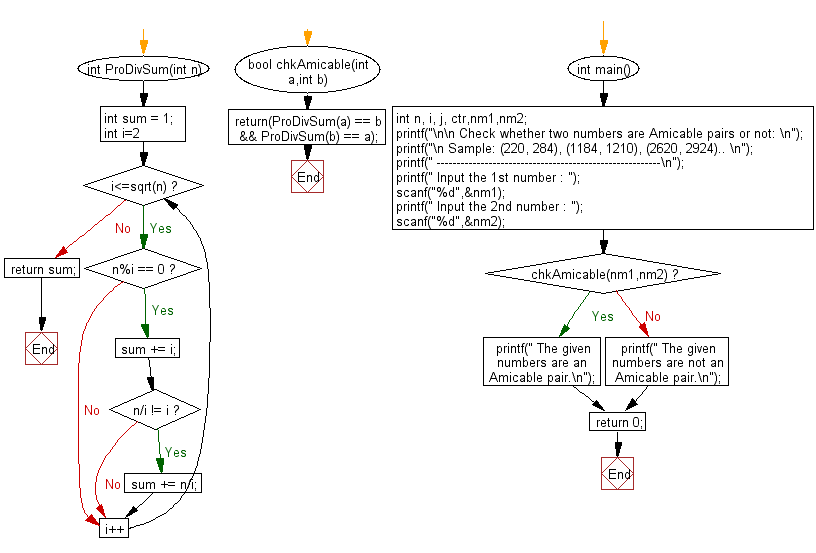﻿ C Program: Check two numbers are Amicable numbers or not - w3resource# C Exercises: Check two numbers are Amicable numbers or not

## C Numbers: Exercise-25 with Solution

Write a program in C to check two numbers are Amicable numbers or not.

Test Data
Input the 1st number : 1184
Input the 2nd number : 1210

Sample Solution:

C Code:

``````# include <stdio.h>
# include <stdlib.h>
# include <stdbool.h>
# include <math.h>

int ProDivSum(int n)
{
int sum = 1;
for (int i=2; i<=sqrt(n); i++)
{
if (n%i == 0)
{
sum += i;
if (n/i != i)
sum += n/i;
}
}
return sum;
}
bool chkAmicable(int a,int b)
{
return(ProDivSum(a) == b && ProDivSum(b) == a);
}
int main()
{
int n, i, j, ctr,nm1,nm2;
printf("\n\n Check whether two numbers are Amicable pairs or not: \n");
printf("\n Sample: (220, 284), (1184, 1210), (2620, 2924).. \n");
printf(" --------------------------------------------------------\n");
printf(" Input the 1st number : ");
scanf("%d",&nm1);
printf(" Input the 2nd number : ");
scanf("%d",&nm2);

if( chkAmicable(nm1,nm2))
printf(" The given numbers are an Amicable pair.\n");
else
printf(" The given numbers are not an Amicable pair.\n");
return 0;
}
```
```

Sample Output:

``` Input the 1st number : 1184
Input the 2nd number : 1210
The given numbers are an Amicable pair.
```

Pictorial Presentation:Flowchart:C Programming Code Editor:

What is the difficulty level of this exercise?

Test your Programming skills with w3resource's quiz.

﻿